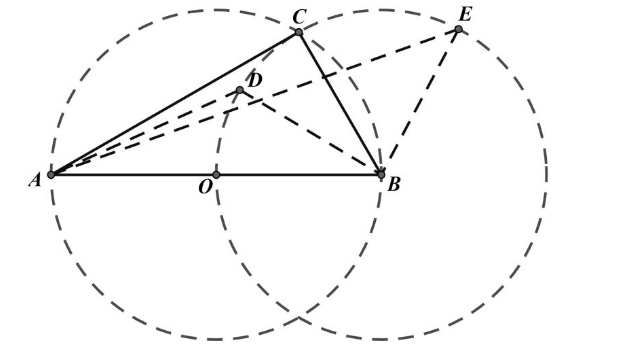【答案】 解: $\angle A=30^{\circ} 。 \angle B=60^{\circ}, \angle C=90^{\circ}$ 显然符合已知条件。

$\angle C=90^{\circ}$ 有 $C$ 在以 $A B$ 为直径的圆 $O$ 上